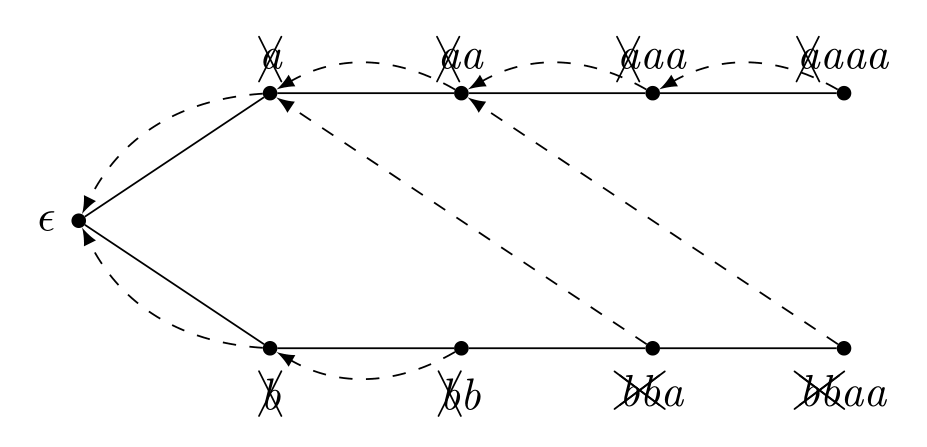# Educational Codeforces Round 71 (Rated for Div. 2) G. Indie Album

https://codeforces.com/contest/1207/problem/G

I'm not good at string problems, but I have solved it luckily. First we construct the Aho-Corasick of the query strings. If N strings is produced by type 2 except the first string, we can directly solve it. When there are branches, we can solve it by the persistent data structure which supports adding value to the specific element and finding the sum of the specific interval. There is a solution doesn't use persistency, but I didn't.

Regarding as Aho-Corasick, the node on Aho-Corasick has a certain edge different from Trie. It connect the string that the longest suffix appears in Trie. The pointer is on a certain node after repeated transitions, we have to increment the count not only this node but also the nodes reachable from it using the special edge. For example, we are on the string bbaa in the following figure, we should add 1 to bbaa, aa, a, and the empty string. These special edges forms a tree. Therefore, we might think add ones between a node and the root. But it requires a complicated structure such as heavy light decomposition, so we want to avoid it. Fortunately, we can calculate the desired value using Euler tour. Both the time and the space complexity is linear logarithmic. When the space complexity is linear logarithmic, we often violates the memory limit. Maybe, lazy propagation segment tree will over the limit.\documentclass[dvipdfmx,margin=2mm]{standalone}
\usepackage{tikz,cancel}
\begin{document}

\begin{tikzpicture}[foo/.style={circle,draw=transparent,fill=black,inner sep=0,minimum size=1mm}]
% {aaaa,bbaa}

\node[foo,label=left:$\epsilon$](0) at (0,0) {};
\node[foo,label=above:\textit{\xcancel{a}}](1) at (1.5,1) {};
\node[foo,label=above:\textit{\xcancel{a}a}](2) at (3,1) {};
\node[foo,label=above:\textit{\xcancel{a}aa}](3) at (4.5,1) {};
\node[foo,label=above:\textit{\xcancel{a}aaa}](4) at (6,1) {};
\node[foo,label=below:\textit{\xcancel{b}}](5) at (1.5,-1) {};
\node[foo,label=below:\textit{\xcancel{b}b}](6) at (3,-1) {};
\node[foo,label=below:\textit{\xcancel{bb}a}](7) at (4.5,-1) {};
\node[foo,label=below:\textit{\xcancel{bb}aa}](8) at (6,-1) {};

\draw (0) to (1);
\draw (1) to (2);
\draw (2) to (3);
\draw (3) to (4);
\draw (0) to (5);
\draw (5) to (6);
\draw (6) to (7);
\draw (7) to (8);

\draw[dashed,-latex] (4) to[bend right] (3);
\draw[dashed,-latex] (3) to[bend right] (2);
\draw[dashed,-latex] (2) to[bend right] (1);
\draw[dashed,-latex] (1) to[bend right] (0);

\draw[dashed,-latex] (8) to (2);
\draw[dashed,-latex] (7) to (1);
\draw[dashed,-latex] (6) to[bend left] (5);
\draw[dashed,-latex] (5) to[bend left] (0);
\end{tikzpicture}

\end{document}